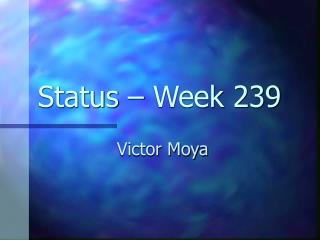DownloadDownload PresentationStatus – Week 239

# Status – Week 239

Download Presentation## Status – Week 239

- - - - - - - - - - - - - - - - - - - - - - - - - - - E N D - - - - - - - - - - - - - - - - - - - - - - - - - - -
##### Presentation Transcript

1. Status – Week 239 Victor Moya

2. Summary • Primitive Assembly • Clipping triangle rejection. • Rasterization. • Triangle Setup. • Early Z. • Current status.

3. Primitive Assembly • Works as a LRU cache. • Asks the Post T&L cache for missing vertex. • Checks if some of the new vertex are already in the primitive assembly cache. • Three vertex stored (2 for triangles, 3 for quads). • Last vertex is always bypassed directly to Triangle Setup.

4. Clipping Rejection • Check clipping per vertex. • Apply results per primitive. • Reject full primitives. • DP3 clip plane equation with vertex homogeneous coordinates. • Signed distance between the vertex and the plane. • Clip the primitive when all the vertex are negative for some of the planes. • Problem: triangles with all vertex outside the clip volume, but with a region inside.

5. Rasterization Primitive Assembly Triangle Setup Traversal Interpolation Setup(vattrib) nextFragment() Interpolate(fr) Rasterizer Emulator

6. Rasterization • Boxes only carry timing. • Latency and throughput for the setup, traversal and interpolation operations. • Rasterizer Emulator performs the actual work: • Setup algorithm. • Traversal algorithm. • Interpolation algorithm.

7. Rasterization • Timing and rasterization algorithm are independent. • Rasterization boxes can simulate as many ‘stages’ as needed without worrying about functionality. • Rasterizer emulator offers an interface for all the rasterization operations: • Setup(), Area(), AreaSign(), GenerateNextFragment(), GenerateNextTile(), InterpolateFragment(), InterpolateFragmentAttribute(), etc…

8. Rasterization • Setup Box: • Get the triangle vertex positions and attributes. • Send to internal signal ‘setup’ -> simulates setup latency. • Read internal signal ‘setup’. • RastEmu::setup(vattrib). • RastEmu::getArea(). • Check area sign and face culling method: • Reject if area is zero or near zero. • Reject if face culling enabled and wrong sign. • Invert coefficient signs if front face culling. • Issue triangle to triangle traversal.

9. Rasterization • Traversal Box: • Read triangles from Setup box. • Set start point: RastEmu::setStart(). • Optional? • Algorithm dependant? • Ask for next fragment/fragment tile: write to internal signal ‘next fragment’. Simulates fragment generation latency. • Read generated fragment: read ‘next fragment’ signal. • RastEmu::nextFragment(). • Send fragment to interpolation.

10. Rasterization • Traversal Box: • Other algorithms could not provide a fragment per cycle or have variable latency for each generated fragment. • RastEmu::nextFragment() could return a boolean. • RastEmu::nextFragment() could return the number of generated fragments (or a mask for a tile). • RastEmu::nextFragment() could return the ‘amount of work’. • Additional interface functions for fragment generation and triangle traversal. • Fragment culling is done in the rasterizer emulator?

11. Rasterization • Interpolation box: • Read fragments from Traversal box. • Interpolate -> write to ‘interpolate’ signal. • per fragment, or • per attribute • Read ‘interpolate’ signal. • RastEmu::interpolate(). • Repeat if per attribute/group of attributes. • Send to fragment FIFO.

12. Triangle Setup • Using hardware equivalent to a vertex shader. • Use multithreading to hide dependecy latencies. • Same as shaders. • Multiple triangles at setup at the same time. • Minimum setup latency: • 6 cycles (just adj(M) using McCool method). • Minimum initialization latency: • 1 cycle using multithreading and enough registers.

13. Triangle Setup • Registers: • rA, rB, rC -> Edge equations a, b and c coefficients (adj(M) and M-1 matrix rows). • rX, rY, rW -> the 3 vertices x, y and w coordinates (M colums). • rD, rI -> matrix determinant and reciprocate. • rR -> 1/w equation coefficients. • rU -> parameter values at the three vertices • rP -> parameter equation coefficients

14. Triangle Setup • Adj(M): (at least 6 cycles + lat. dep.) mul rC.xyz, rX.yzx, rY.zxy mul rB.xyz, rX.zxy, rW.yzx mul rA.xyz, rY.yzx, rW.zxy mad rC.xyz, rX.zxy, rY.yzx, -rC mad rB.xyz, rX.yzx, rW.zyx, -rB mad rA.xyz, rY.zxy, rW.yzx, -rA

15. Triangle Setup • det(M): (1 cycle) • M-1: (4 cycles + dep. lat.) dp3 rD.x, rC, rW rcc rI.x, rD.x mul rC, rC, rI mul rB, rB, rI mul rA, rA, rI

16. Triangle Setup • 1/w coefficients: (2 cycles + dep. lat.) • Parameter coefficients: (3 cycles) add rR, rA, rB add rR, rR, rC dp3 rU.x, rP, rA dp3 rU.y, rP, rB dp3 rU.z, rP, rC

17. Early Z • Could be implemented before interpolation. • Interpolate the triangle Z (z/w) first. • Could save some calculations. • Would save time?

18. Current Status • (to be done)